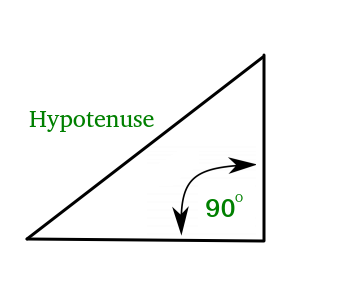PHP | hypot() function

The hypot() function is a built-in mathematical function in PHP and returns the square root of sum of square of arguments passed. It finds the hypotenuse, hypotenuse is the longest side of a right angled triangle. It is calculated by the formula :where x and y are the other two sides of the triangle.Syntax:

hypot(x,y);

Parameters used:

• x : the length of first side.
• y: the length of the second side.

Return Type: The return type of hypot(x,y) is Float and it returns the square root of squares of values passed to it as parameters.

Examples:

Input : x=3, y=4
Output :5

Input :x=9, y=10
Output : 13.453624047074

Below program illustrates the working of hypot() function in PHP:



Output:

5
7.211102550928

Related Article: hypot() in C++

My Personal Notes arrow_drop_upCheck out this Author's contributed articles.

If you like GeeksforGeeks and would like to contribute, you can also write an article using contribute.geeksforgeeks.org or mail your article to contribute@geeksforgeeks.org. See your article appearing on the GeeksforGeeks main page and help other Geeks.

Please Improve this article if you find anything incorrect by clicking on the "Improve Article" button below.

Article Tags :
Practice Tags :

Be the First to upvote.

Please write to us at contribute@geeksforgeeks.org to report any issue with the above content.